##MSIPO技术圈 首页 IT技术 查看内容

2023-07-13

# 步骤

## 1、在服务器上安装open3d

``````pip3 install open3d
``````

## 3、配置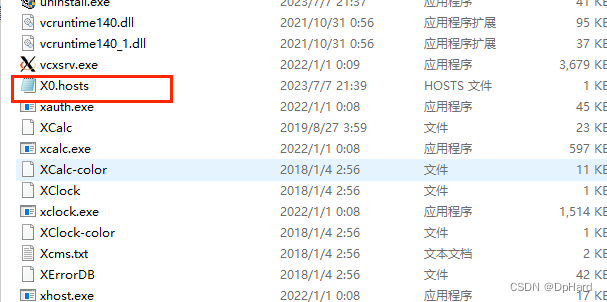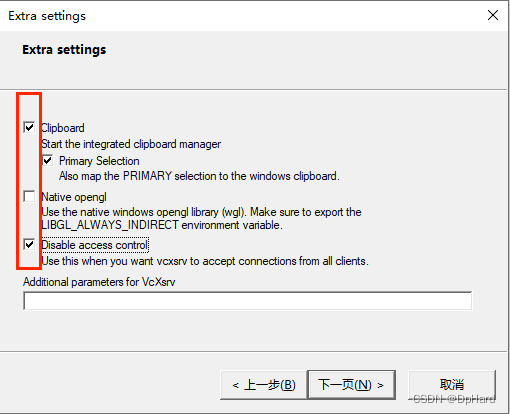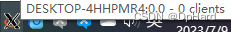## vscode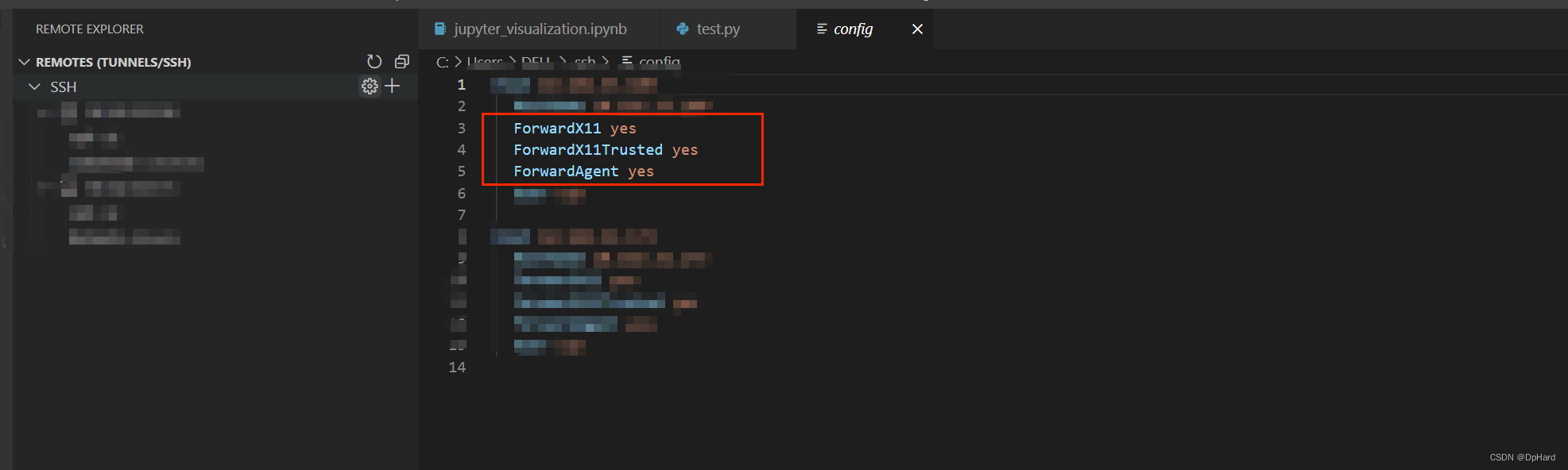## 远程服务器

`````` export DISPLAY="本机ip:端口号"
``````

``````echo \$LIBGL_ALWAYS_INDIRECT
``````

## 运行测试程序

``````import numpy as np
import open3d as o3d

points = np.random.rand(10000, 3)
point_cloud = o3d.geometry.PointCloud()
point_cloud.points = o3d.utility.Vector3dVector(points)
o3d.visualization.draw_geometries([point_cloud])
``````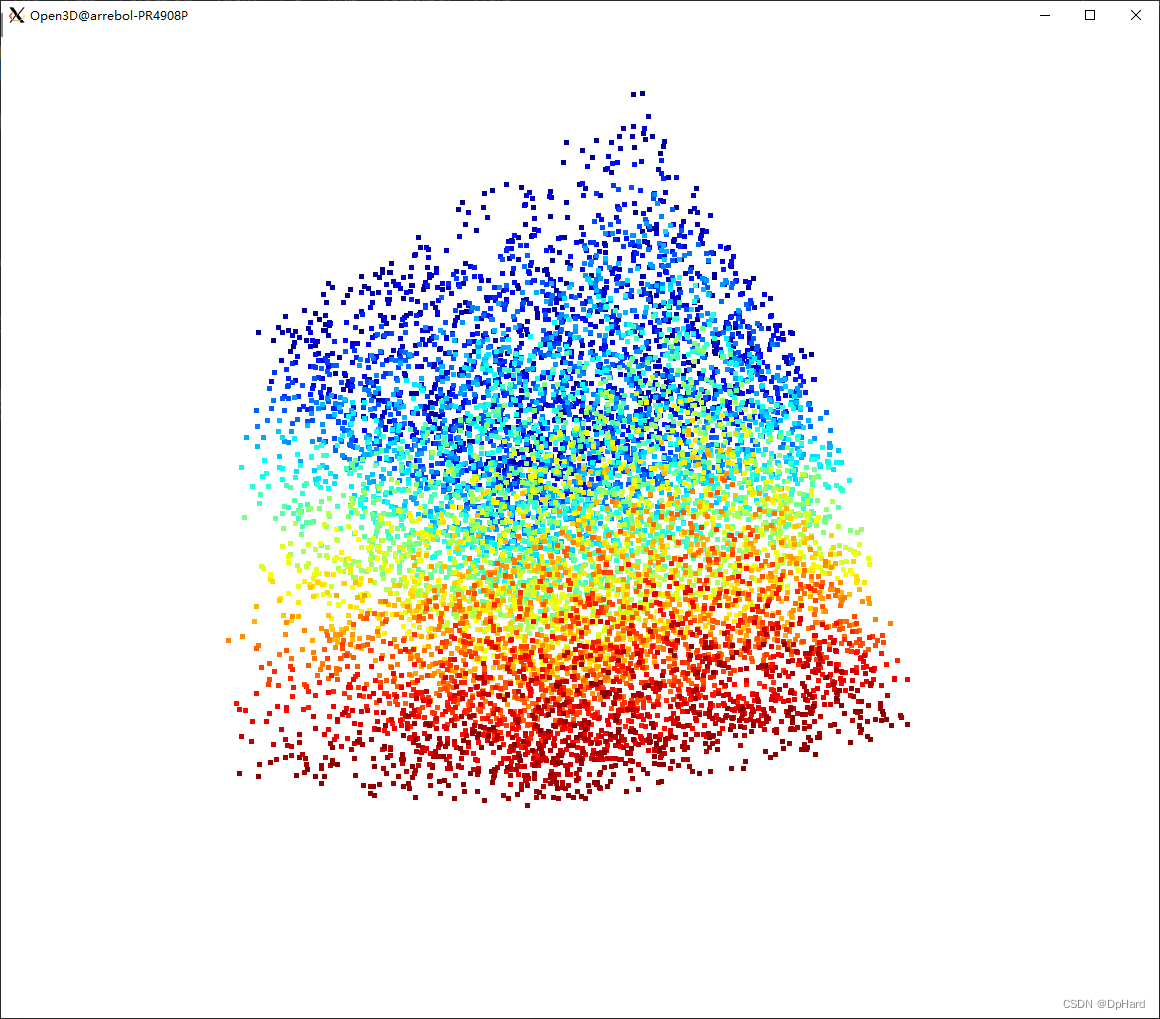# 参考资料

https://github.com/isl-org/Open3D/issues/897

# 可能的其他解决方案

1、web可视化
web可视化

``````import open3d as o3d
from open3d.web_visualizer import draw

cube_red = o3d.geometry.TriangleMesh.create_box(1, 2, 4)
cube_red.compute_vertex_normals()
cube_red.paint_uniform_color((1.0, 0.0, 0.0))
draw(cube_red)
``````